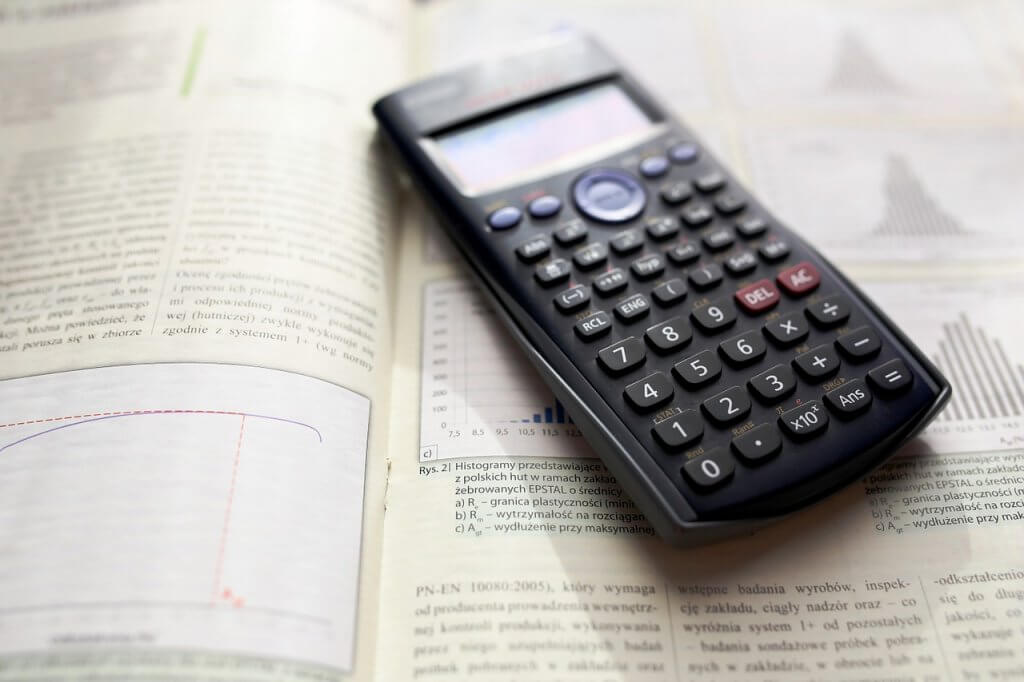# Measures of central tendencies and dispersion

Mean, mode, and median are referred to as measures of central tendencies while measures of spread include standard deviation, range, and variance. Most of us are familiar with the mean since it is prevalent as compared to the rest. But first things first, so what is the meaning of central tendency? Central tendency is a branch of descriptive statistics. A measure of central tendency is a single value that tries to explain a data set by pointing out the middle point within the dataset. Measures of spread or dispersion, on the other hand, determine how close or far a set of data is from the mean. Well, let’s take a look at these measures and study how to calculate them.

## Mean

Also known as average. It is the addition of all the values in a data set divided by the total number of objects in a data set. Mean can be determined for both continuous and discrete data. The mean represents the most common value. One drawback is that values that are either too small or too large as compared to the rest in the set influence the mean. Various means exist, such as arithmetic, sample mean, etc.

## Median

Median is the central value in a collection of data. First, the data is arranged from smallest to largest before the median can be determined. The number that is in the middle of the data set is usually the median.

## Mode

In a scenario where you have a collection of data or a representation of data using a histogram, the mode is the most frequent value in the data set or the highest bar in the histogram. When we have more than two values that appear on many occasions, it becomes challenging to determine the mode of that dataset.

## Range

The range is the most common and easily understood method of dispersion. It is the difference between the maximum and minimum value in a set of data. Data is arranged in ascending order, and after that, the highest number is subtracted from the lowest one.

## Variance

Variance is calculated by deducting the mean from every value in a data set and squaring each value then dividing it by the total number of available objects in a set. Used to give a general overview of the data.

## Standard deviation

Standard deviation is closely related to the mean. That is evident from the computation of the standard deviation. It is another method of dispersion. Tells us how numbers distribute about the mean.it is obtained by getting the square root of the variance.

### Factoring

It is the process of splitting an expression into simpler expressions. Numbers have factors; these factors can be interpreted down into their more straightforward form. Factoring is done to a complicated algebra equation until no more factoring is possible.

For a student taking statistics, these are some of the most common methods of describing a set of data; they will encounter in their education journey. Therefore, it is in their best interest to familiarize themselves with them because, as they continue, other complicated measures can emerge that require skill in these measures. But in case you’re having some trouble with them, be sure to let us know. We offer website that answers math problems to a student at various levels of study. Can I pay for homework? Yes.